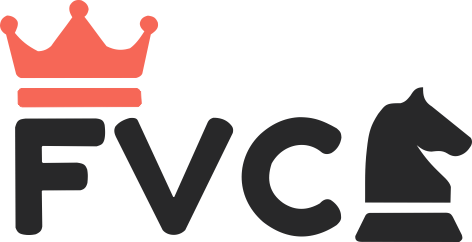## Foundation

1 session per week x 60 min

Program includes:

Number Sense and Operations:
• Counting and basic operations (addition and subtraction in early grades, multiplication and division introduced in later grades).
• Place value understanding.

Fractions, Decimals, and Place Value:
• Introduction to fractions.
• Place value up to thousands.
• Decimal and fraction operations (G4).

Measurement and Geometry:
• Time-telling skills (hour, quarter-hour, and minute).
• Measurement of length, capacity, and weight.
• Basic geometry and shape recognition.

Data Analysis and Probability:
• Data collection and graph interpretation.
• Introduction to statistics (mean, median, mode in G3).
• Basic probability concepts (G4).

## Classwork

1 session per week x 90 min

Program includes:

Math Fundamentals:
• Comprehensive understanding of addition, subtraction, multiplication, and division.
• Introduction to advanced operations, including algebraic concepts.

Numbers and Decimals:
• Mastery of fractions and decimals, along with operations.
• Proficiency in place value, up to millions.

Measurement and Geometry Mastery:
• Strong measurement skills, including conversions and complex shapes.
• In-depth exploration of geometry, covering properties, volume, and trigonometry.

Data Analysis and Probability Skills:
• Proficient data analysis and interpretation skills.
• Advanced statistics and probability calculations.
• Applying mathematical concepts to solve complex real-world problems.

## Competition

1 session per week x 90 min

Program includes:

Algebra and Equations:
• Master linear, quadratic, and polynomial equations.
• Understand systems of equations.

Geometry and Trigonometry:
• Grasp Euclidean geometry principles.
• Learn basic trigonometry and coordinate geometry.

Number Theory and Combinatorics:
• Exploring prime numbers, divisibility, and counting principles.
• Understanding modular arithmetic and probability.

Problem Solving:
• Practice with challenging problems, focusing on Olympiad-style questions.

Practice Exams:
• Regular Practice exams to simulate competition conditions.

Resources and Support:
• Utilize advanced math textbooks and resources.
• guidance from mentors or teachers with competition experience.

#### Program Overview

The Math Program is designed to provide students with a comprehensive understanding of mathematical concepts and skills necessary for success in competitions. The program aims to foster a deep appreciation for mathematics, promote critical thinking, and build problem-solving abilities.

• Curriculum Coverage: The program covers a wide range of math topics, including [list key topics or areas of focus].
• Interactive Learning: Engage in interactive lessons, discussions, and hands-on activities to reinforce understanding.
• Assessments: Regular assessments and quizzes to track progress and identify areas for improvement.
• Real-World Applications: Explore practical applications of mathematics in everyday life and various fields.
• Problem-Solving Skills: Develop strong problem-solving skills through challenging math problems and puzzles.
• Individualized Support: Personalized assistance and support for students who need extra help.
• Peer Collaboration: Collaborative group activities to encourage teamwork and learning from peers.
• Math Competitions: Preparation for math competitions to challenge and expand mathematical abilities.

## Fraser Valley Chess Club Registration

Membership Details - Step 1 of 2